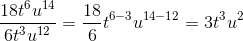# ISEE Upper Level Math : How to divide exponents

## Example Questions

1 3 Next →

### Example Question #101 : Numbers And Operations

Simplify: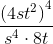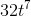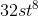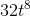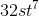Explanation: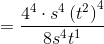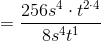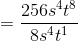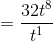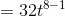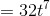### Example Question #51 : Exponential Operations

Simplify the following expression: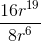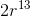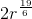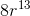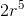Explanation:

Simplify the following expression:To solve this question, we need to recall that when dividing exponents we subtract them.

When dividing coefficients, we treat them as regular division.

In this case, we can break up our question into two parts: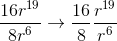The coefficients will simply reduce to "2", because 16 divided by 8 is two

The r's are another story. We subtract, so we will get the following: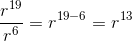So, if we put our two parts back together, we get:### Example Question #103 : Numbers And Operations

Simplify the following: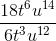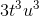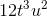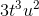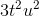Explanation:

Simplify the following:Let's begin by recalling that when dividing variables with similar base, we need to subtract the exponents.

To deal with the coefficients, simply treat them as a fraction and simplify.